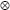Technical Details >  Modes of Operation

## Modes of Operation

The mode of operation used by VeraCrypt for encrypted partitions, drives, and virtual volumes is XTS.

XTS mode is in fact XEX mode , which was designed by Phillip Rogaway in 2003, with a minor modification (XEX mode uses a single key for two different purposes, whereas XTS mode uses two independent keys).

In 2010, XTS mode was approved by NIST for protecting the confidentiality of data on storage devices . In 2007, it was also approved by the IEEE for cryptographic protection of data on block-oriented storage devices (IEEE 1619).

Description of XTS mode:
Ci = EK1(Pi ^ (EK2(n)ai)) ^ (EK2(n)ai)
Where:denotes multiplication of two polynomials over the binary field GF(2) modulo x128+x7+x2+x+1 K1 is the encryption key (256-bit for each supported cipher; i.e, AES, Serpent, and Twofish) K2 is the secondary key (256-bit for each supported cipher; i.e, AES, Serpent, and Twofish) i is the cipher block index within a data unit;   for the first cipher block within a data unit, i = 0 n is the data unit index within the scope of K1;   for the first data unit, n = 0 a is a primitive element of Galois Field (2128) that corresponds to polynomial x (i.e., 2) Note: The remaining symbols are defined in the section Notation.

The size of each data unit is always 512 bytes (regardless of the sector size).
For further information pertaining to XTS mode, see e.g.  and .

Next Section >>